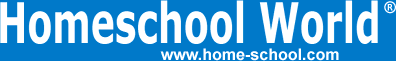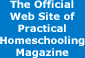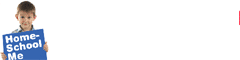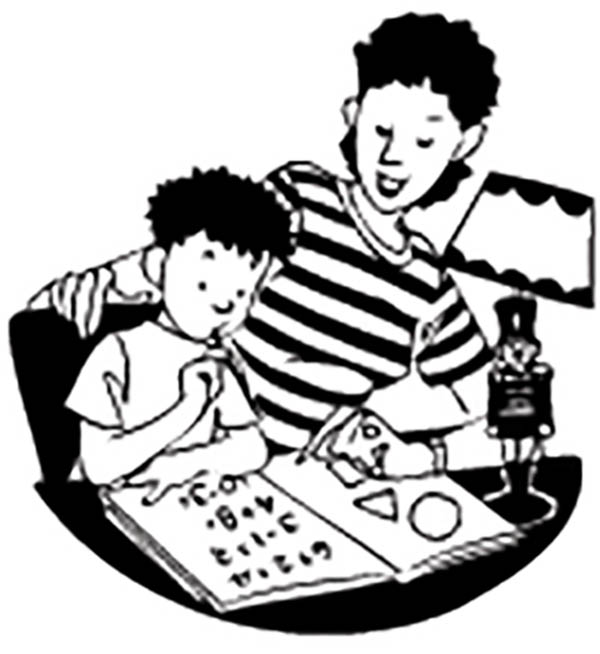Home Curriculum Catalog Articles Contests Events Groups Forum News ContactGetting Started Preschool/K Elementary Middle School High School College & Career Prep Special Needs Group Activities Research FAQs# Lies, Part III: The Importance of Consistent Verbalizations

By Bob Hazen
Printed in Practical Homeschooling #19, 1997.Don't get fractured over fractions.This is the third and final article in a series about the importance of teaching mathematical principles in a consistent manner.

In the past two articles, I have claimed that certain math verbalizations (spoken explanations) are lies. By lies, I mean statements that are sometimes simply wrong or, more often, only true within a very limited context.

Let's take the subtraction exercise 72 - 38; if we say, "You can't take 8 from 2 . . ." we are not being truthful with students, since anyone who's bounced a check (\$2 - \$8 = -\$6) or lived in a cold climate (2° - 8° = 6° below zero) knows that 2 minus 8 is -6. The verbalization "You can't take 8 from 2 . . ." is only true within the limited context of the "Physical removal" model of subtraction (for example, doing a regrouping exercise when working with manipulatives). "Physical removal" isn't the only way to represent subtraction, yet too often it's the only explanation children hear.

My emphasis here is that the "big picture" of what comes later in life and in mathematics instruction should guide the particular details of how we explain math to children in the elementary years. Our explanations need to be accurate to the mathematical facts, concepts, and activities they are describing. They also should be consistent with other modalities and with other math topics in later grades. Whenever possible, the words we use (the Verbal mode) should be consistent with the symbols (the Abstract mode), the manipulatives (the Concrete mode), and the sketches (the Pictorial mode) that our children also work with. When there is inconsistency or contradiction among these modes, kids can get confused and frustrated.

Let's examine one last inconsistent verbalization regarding the four basic arithmetic operations.

Example #7

The Lie: Adding and multiplying make more. Subtracting and dividing make less.

The Problem: If adding makes more, then how can we start with 7, add -2, and get the smaller amount of 5 (7 + -2 = 5)? If multiplying makes more, then why does 1/4 x 1/3 = 1/12, where 1/12 is smaller than either the 1/4 or the 1/3? If subtracting makes less, then how can I have \$50 as my checkbook balance, remove a previous incorrect overdraft charge of \$10, and end up with a balance of \$60, which is more than what I started with (50 - -10 = 60)? If dividing makes less, then if I want to divide 2 of my dollar bills into quarters, how do I get 8 quarters, which is larger than either the 2 or the 1/4 (i.e., 2 / 1/4 = 8)?

The Why: Adding makes more only if we are adding a positive number to whatever amount we start with. Subtracting makes less only if we are taking away a positive number. Multiplying makes more only if the multiplier is greater than 1. Dividing makes less only if the divisor is greater than 1.

The Confusion Now: Since traditional K-4 math almost exclusively uses positive whole numbers to teach the four basic operations, there's usually not much confusion - yet. Nonetheless, inconsistent verbalizations in these grades are too often setting kids up for confusion in later grades.

The Confusion Later: Upper-elementary math deals with multiplying and dividing by fractions less than 1, and junior-high math begins to deal with adding and subtracting negative numbers. Students who have been taught the lie that "adding and multiplying make more, and subtracting and dividing make less" invariably get confused by fifth grade exercises like 1/2 x 1/3 = 1/6 or seventh grade exercises like 7 - -2 = 9. Students can often see these apparent inconsistencies (in their eyes) as one more way in which "math just doesn't make sense."

The Truth: A truthful generalization is simply that adding and multiplying are joining operations, while subtracting and dividing are separating operations. With the addition exercise 3 + 4, we are joining 3 and 4 to obtain 7 (3 + 4 = 7). With 3 + -4, we are joining these two amounts to get -1 (3 + -4 = -1). With the subtraction exercise 7 - 2, we start with 7 and separate out 2, leaving 5. With the similar subtraction exercise 7 - -2, we start with 7 and separate out (or remove) a debt, which makes our final amount more than what we started with (7 - -2 = 9). With 8 x 2, we are joining units 2 times, which yields 16. With 8 / 2, we are separating 8 into groups of 2, which yields 4.

Note that when we multiply or divide by a simple fraction, the joining or separating is more difficult to verbalize consistently. For exercises like 4 x 1/3, or 1/4 x 1/3, or 8 / 1/2, I go back to the rectangle model as the best means of modeling the dynamics of these situations [PHS #14, p. 20; PHS #15, p. 22]

Consistency is Important in Mathematics . . . and Life

As teachers and parents, we have a godly responsibility to be truthful and accurate in the words we choose for what we are trying to say. When using more than one kind of math model (Real, Verbal, Concrete, Pictorial, Abstract), we have a godly responsibility to use these various models in such a way as to be as harmonious and consistent as possible.

There should be substantial consistency between what we say, see, read, write, and draw mathematically. My experience is that if consistency is lacking, it's usually because I have chosen poor wording to explain what is happening with the manipulatives, the drawings, the symbols, or the procedures being taught. I've learned to examine much more carefully the words I use, in order to make my words fit more consistently - yet still truthfully - with what else is being done. Words and truth matter in all things. Words and truth matter in math.Terms of Use   Privacy Policy Copyright ©1993-2023 Home Life, Inc.